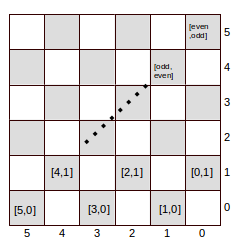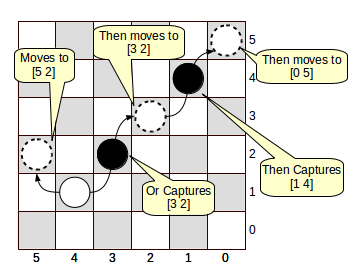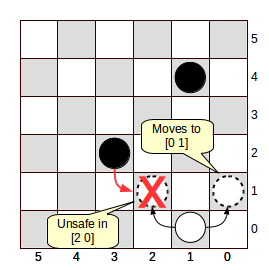# .css-4zleql{display:block;}Tech NovelsRafik Naccache
·Aug 25, 2020·

# Logic Programming: A forgotten Field in AI

Nowadays, when you hear "AI," you are probably going to think about neural networks and model training, starting to reach for data sets and envisioning how to feed them into the learning machine. In this post, I will try to revive a more or less forgotten way to do things—the Logic programming way.

Logic programming is an exciting paradigm, according to which one expresses programs in terms of relations involving some logic variables, and expects results reflecting what do these logical variables have to be so to make sure those relations are verified(Pfeww!).

We are so talking about "goals" that we want to succeed: We want those relations to be actually occurring, and search for the values of our logic variables that would make that happen.

You can see how this sort of programming - also known as relational programming - lays the foundation of an alternative way of approaching computing. It's a declarative method in which you don't explicitly specify how to process inputs into outputs, but where you instead express queries about logical variables arranged in a set of desired relations.

For example, consider the following logical function :

``````(multiplio x y z)
``````

that stands for a goal (or a relation) succeeding if z equals x times y. It will succeed if you query for its result while you specify a relevant value for all of the three logical variables:

``````(multiplio 2 3 6) ;=>Success
``````

But if you specify that you want to know for which values of z it will succeed, specifying actual, fixed values for x and y, you are sort of multiplying two numbers:

``````(multiplio 2 3 q) ;=> Success for q = 6
``````

More interestingly, you can ask what might the value of x be given that y is set to 2 and that the product z at 6

``````(multiplio q 3 6) ;=> Success for q = 2
``````

The previous examples showed how you can write programs in terms of relations, or logic goals, and specify or ask for the values of the variables you please: No inputs, no outputs, just relations.

Of course, when it is unable to make your goals succeed, the relational kernel will give you "nothing." On the other hand, if it notices that the goals are achieved every time, it returns a particular value that says, well, everything.

In the following, we will use relational programming to create an AI solving a round of checkers game. We will use a particular language for logic programming, miniKanren, through its Clojure implementation, core.logic.

# A core.logic Primer

In core.logic, you run your queries using a special interface, run like in the following simple example:

``````(run* [q]
(== q 1))
``````

That is, you're asking core.logic to run a query over the logical variable q, so that the goal (== q 1) is verified. == is miniKanren's unification, that is, the mecanism that makes a goal who succeeds if the terms involved are the same, which sets q to (1) in our particular example. Unification is a very important operation in logical programming, and is implemented up to the level of nested Clojure datastructures:

``````(run* [q]
(== [q 2][1 2]))
``````

yields (1), as we must have q set at 1 so we have the first vector as [1 2] in order to have it unified with the second term. But the careful reader may wonder: why do we have results laid out in sequences? This is beause the logical variable we are querying over might happen to take multiple possible values; as a matter of fact, you can choose how many solution do you want core.logic to go up to. We did mention run* (star) in our query because we want all of the solutions. If we did only want to uncover first five of them, we could have emitted a query like so:

``````(run 5 [q]
(== [q 2][1 2]))
``````

# Playing a Checkers round

Enough talk and let's play checkers. For this, we are going to implement an artificial intelligence kernel capable of analyzing some checkers' board, determining – under the game rules – where to move while staying out of the reach of its opponent's attacks, and spotting when it can capture some of the enemy's soldiers. Let's go through a little remainder of the game of checkers. You and your opponent evolve on a square chessboard, but you can only move on diagonals over black squares one step at a time. Also, you can only move forward. Both of you can capture an enemy piece if it is situated just one step away from one of your pieces, provided that when you jump over it following the diagonal, the black square where you're landing is empty. If, while doing so, from that new position, you can capture another piece, you may go on.

# Modeling the Game

To model the battle scene, we are going to consider three sets: one for the empty positions, one for the only piece that belongs to the checkers' solver engine, and one for the enemy's locations. To describe a particular situation, you may tell the solver what's in each of these sets. As far as the items that will be put in them are concerned, we will store in them coordinates of positions on the checker's board. For instance, to tell that the enemy has 3 pieces, we just throw the 3 vectors representing the positions where these pieces are in the enemy's set.

The following figure explains how we are going to represent the different positions on a chessboard of 6 squares edges. Note that for its construction, to consider only the black squares, we are going to alternate a set of vectors crossing even with odd coordinates, then odd with even ones.The solver is concerned about three significant matters when trying to figure out the action it must take:

1. Where to put the checker,
2. Will the moving piece be captured when it goes there,
3. Whether it can win one or more of its opponent's pieces if after jumping, he has the opportunity to do so. Let's implement it.

## Building the Chessboard

For starters, we are going to declare our ns. Note that we are using the finite domain constrains engine, clojure.core.logic.fd, to be able to derive goals where algebraic operations are involved:

``````(ns recipe19.core
(:require [clojure.core.logic :as logic]
[clojure.core.logic.fd :as fd]))
``````

We now focus on constructing the chessboard. As we mentioned earlier, we will build a set of vectors, associating odd x coordinates with even y ones, then the other way around: even x coordinates with odd y ones:

``````(defn evenso
[size out]
(logic/fresh [x2 se]
(fd/in x2 (fd/interval 0  (/ size 2)))
;; We construct a set of integers
;; going from 0 to half the size
;; using a core.logic finite domain
;; facility
(fd/* x2 2 se)
;; we construct the even coords
(logic/== out se)))
;; and we output them by unifying them
;; with output

(defn oddso [size out]
(logic/fresh [x2 seo so]
(fd/in x2 (fd/interval 0  (/  size 2)))
;; Same logic applies for odd part
(fd/* x2 2 seo)
;; We generate an even number
(fd/+ seo 1 so)
;; Then we add 1 to it
(fd/<= so size)
;; We ensure that we do not exceed
;; size
(logic/== out so)))
;; And we unify with output

(defn boardo [size out]
(logic/fresh [e o]
(oddso size o)
(evenso  size e)
;; Then starting from even and odd numbers
(logic/conde
[(logic/== out [e o])]
;; We combine a first set of vectors
;; Crossing [even odd]
[(logic/== out [o e])])))
;; And crossing [odd even]
``````

## Moving the piece

Let's build the goal function that determines where our piece shall go starting from a current position. This moves our piece up, incrementing the y part of the coordinates, and go to the left and to the right by adding or deducing 1 from the x component:

``````(defn where-to-movo
[empty cur-pos out]
(logic/fresh [->x ->y curx cury]
(logic/== [curx cury] cur-pos)
;; We destructure the position into
;; x and y parts
(logic/conde
[(fd/+ 1 curx ->x)]
[(fd/- curx 1 ->x)])
;; We go left or right,
;; adding or substracting 1
;; to x and storing it into ->x
(fd/+ cury 1 ->y)
;; We can only move up, adding 1 to y
;; and storing it into ->y
(logic/membero [->x ->y] empty)
;; We verify that the target position
;; is empty by seeing if it is present
;; in the empty set
(logic/== out [->x ->y])))
;; And we unify with the output
``````

## Don't Get Captured!

After the solver has determined where it can move its piece, it needs a goal function that tells if, at that position, that piece won't be captured by any of the opponent's pieces. That's the aim of the following goal function:

``````(defn isnt-capturedo
[empty mypos enemy out]
(logic/fresh [x->
y->
->x
->y
y->y
x->x
new-x
new-y]
(logic/== [x-> y->] enemy)
(logic/== [->x ->y] mypos)
;; We destructure enemy and our
;; position
(logic/conde
[(fd/- x-> ->x x->x)]
[(fd/- ->x x-> x->x)])
;; As core.logic.fd can't handle
;; negative numbers, we must use
;; a conde so we can get the difference
;; between the coords, whether is enemy's x higher
;; than the solver's, or the other way around.
(fd/- y-> ->y y->y)
;; We know that the opponent can only go down
;; so we are able to proceed with a single
;; substraction, that is the opponent's y minus the
;; solver's
(logic/conde
[(fd/== x->x 1)
(fd/== y->y 1)
;; This enemy's piece is close
;; enough to the solver's, let's check
;; if it can capture it.
(logic/conda
[(fd/> x-> ->x)(fd/- ->x 1 new-x)]
;; Where is the landing x coord had
;; the enemy captured the solver's
;; if it is at its left?
[(fd/< x-> ->x)(fd/+ ->x  1 new-x)])
;; And where would it be if it did come
;; feom the right?
(fd/- ->y y->y new-y)
;; What would the landing position y be, after
;; capture of the solver's piece?
(logic/nafc logic/membero [new-x new-y] empty)
;; If it is not empty, the solver's piece is
;; safe
(logic/== out true)]
[(fd/> x->x 1)(logic/== out true)]
;; The difference between coordinates
;; on the x axis is higher than 1,
;; This piece isn't a threat to us
[(fd/> y->y 1)(logic/== out true)])))
;; Same logic for the Y axis
``````

## Catch'em all!

Our artificial checkers' player needs a goal function to help him spot the opportunity of capturing some enemy pieces. This is a recursive process, as after jumping over the piece it captured, we need to check if there is something else to recapture. That's why we built a tabled goal, that ensures termination by "memoizing" the different values taken by the tabled logical variables and deciding to exit en execution whenever the same patterns are encountered over and over again:

``````(def enemy-piece-capturo
(logic/tabled
;; A tabled goal ensures that our
;; recursive goal terminates by
;; detecting recurrent patterns
;; among the values of the logical
;; variables
[empty pos->
enemies
out]

(logic/fresh [x->
y->
->x
->y
x->x
y->y
new-x
new-y
new-path]
(logic/== [x-> y->] pos->)
(logic/membero [->x ->y] enemies)
;; We destructure our piece's and
;; all of the enemies' positions
(logic/conde
[(fd/- x-> ->x x->x)]
[(fd/- ->x x-> x->x)])
;; As negative numbers are considered
;; as failing goals, we yield a union
;; of both cases to be sure to get a
;; difference in x->x
(fd/- ->y y-> y->y)
;; We know that this is our piece,
;; we can only go up
(logic/conde
[(fd/> x-> ->x)(fd/- ->x 1 new-x)]
[(fd/< x-> ->x)(fd/+ ->x  1 new-x)])
;; the new-position's x after jumping,
;; adding +1 or -1 to the captured piece's x
;; depending of whether
;; we are going right or left
(fd/+ ->y y->y new-y)
;; New y can only be the enemy's piece incremented
;; by 1 as we go always up.
(logic/membero [new-x new-y] empty)
;; This landing position must be empty
(fd/== x->x 1)
(fd/== y->y 1)
;; And we must be exactly one square away
;; from the opponent's captured piece
(logic/== new-path [:from [x-> y->]
:capture [->x ->y]
:then-> [new-x new-y]])
;; At this point we have a new path:
;; Where we started, what did we capture
;; and where we landed
(logic/conde
[(logic/== out  new-path)]
;; we emit this new path,
;; as if we were to stop here,
;; this is considered a legal move.
[(logic/fresh [np]
(enemy-piece-capturo empty
[new-x new-y]
enemies
np)
;; And we recursively check for another possible
;; piece to capture, adding any new discovered
;; path to what we've captured so far
(logic/conjo [new-path :then->] np out))]))))
``````

## Sticking it all together

With all the building blocks for the chessboard analyzer ready, we can design a goal function that plays a round of checkers, minding all of the three matters constituting the engine's life: moving, keeping away from being captured and capturing enemy's soldiers:

``````(def play-roundo
(logic/tabled  [empty
cur-pos
enemies
out]
;; This is a tabled goal so we can
;; avoid some redundancy in the results
;; caused by the unification engine
(logic/fresh [new-pos
new-empty
not-captured?
captured-them]
(logic/conde
;; A main conde combining two main moves:
;; 1. Capturing and emitting what has been
;; captured
[(enemy-piece-capturo empty
cur-pos
enemies
captured-them)
(logic/== out captured-them)]
;; 2. Moving while keeping safe.
[(where-to-movo empty
cur-pos
new-pos)
(logic/conjo empty cur-pos new-empty)
;; After moving, the piece former position
;; is empty
(logic/everyg  #(isnt-capturedo new-empty
new-pos
%
not-captured?) enemies)
;; All of the enemy's pieces must not be able to
;; capture our piece once it moved to
;; its new position
(logic/== out [:move-> new-pos])]))))
;; and we emit the positions in which we are safe.
``````

# The Checkers player in Action

## Initializing the game

To be able to give some checkers' puzzles to the solver, we need an initialization function, that takes one set of enemies, the engine's piece, and emits the right set of empty squares, that is, the whole board minus the two first set:

``````(defn prepare-board
[board-size enemies me]
(let [initial-board (set  (logic/run* [q]
(boardo
board-size
q)))]
;; We generate a board of size board-size
(into '()  (clojure.set/difference initial-board (set
(conj
enemies
me))))))
;; and we emit empty pieces: the whole starting set minus
;; enemy and "me" pieces
``````

## First Challenge: Multi-Capture

Consider the situation depicted by the following figure:The solver can safely move left or capture one or two pieces. Let's submit this situation to the solver by issuing at your REPL:

``````(let [enemies '([1 4][3 2]) ;; positioning the enemies
me [4 1]
empty-brd (prepare-board 8 enemies me)]
(logic/run* [q]
(play-roundo
empty-brd
me
enemies
q)))
``````

The results are the following:

``````([:move-> [5 2]] ; the simple move

[:from [4 1] :capture [3 2] :then-> [2 3]] ; one capture

[[:from [4 1] :capture [3 2] :then-> [2 3]] ; two captures
:then->
[:from [2 3] :capture [1 4] :then-> [0 5]]])
``````

## Second Challenge: Staying safe

Consider the following situation:Although our piece can be moved left or right, the solver won't take the first choice as the enemy will capture the piece at [2 0]. To see it in action, type at the REPL:

``````(let [enemies '([3 2][1 4]) ; positioning the enemies
me [1 0]
empty-brd (prepare-board 8 enemies me)]
(logic/run* [q]
(play-roundo
empty-brd
me
enemies
q)))
``````

And the solver chooses:

``````([:move-> [0 1]])
``````

# Conclusion

We've seen how it is possible to tackle the checkers' problem using the relational programming paradigm. We used a clojure based implementation of the minikanren relational programming framework. We saw how we could model three of the game constraints: moving, staying safe, and trying to take prisoners.

On a final note, please be aware that this approach requires you to know what you're doing. As it is operating searches on deep forests of possible solution combinations, you must know how to model your program and be sure you don't hit resource limits if you throw in un-manageably sized problems. This been said, there's a significant body of knowledge concerned about mastering this kind of problem search. So, if you want to take this to production, you definitely need to do your homework and learn this art thoroughly.

This is inspired by a chapter of my Book Clojure Data Structures and Algorithms Cookbook